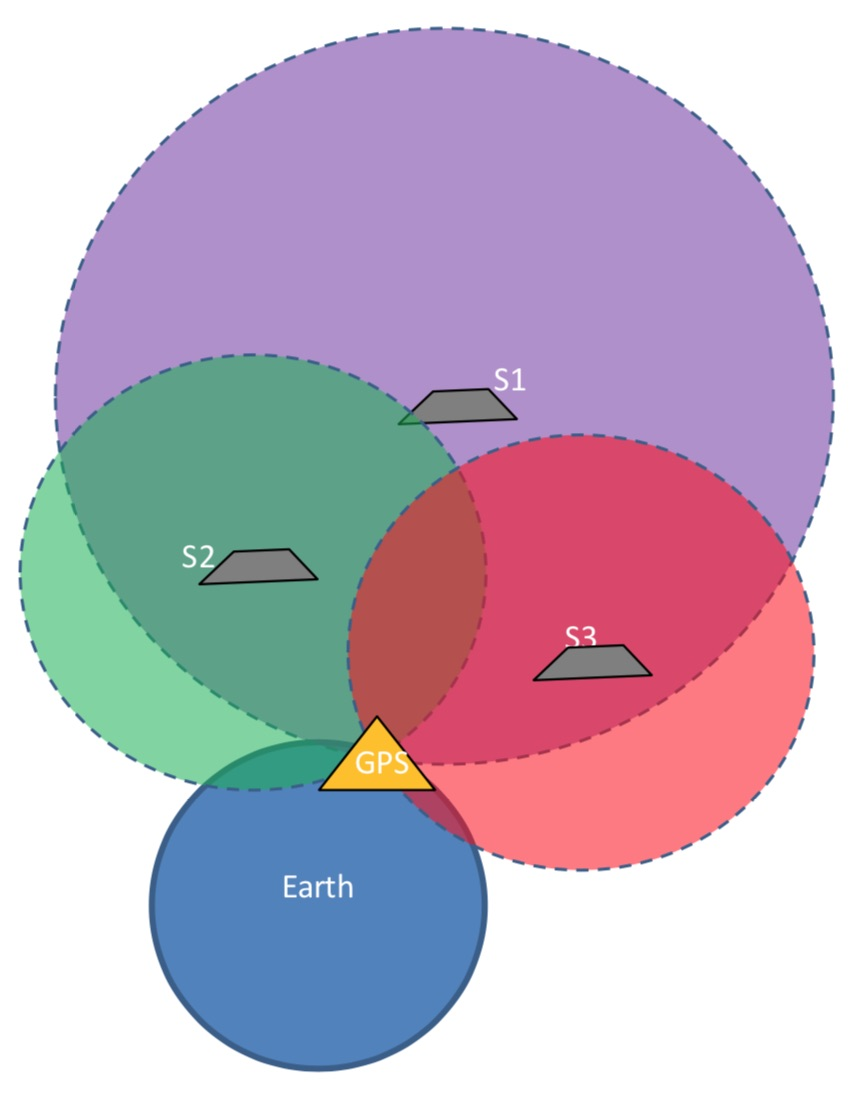Feb 26, 2016

Feb 26, 2016

Feb 26, 2016

# GPS Constellation and Characteristics of GPS ConstellationA Satellite System used to pinpoint a Geographic Location of a user’s receiver anywhere in the world

Two GNSS systems are currently in operation:

• GPS (United States’ Global Positioning System)
• GLONASS (Russian Federation’s Global Orbiting Navigation Satellite System)

Other GNSS under Development:

• Galileo Positioning System (European Union) (2020)

## Satellite Constellation

A group of artificial satellites working in concert The satellites are:

• Synchronized
• Operate together under shared control

## Characteristics of GPS Constellation

Following are some of the characteristics of GPS constellation:

1. 24 Satellites
2. 6 Orbits
3. 4 Satellites per orbit
4. Orbit Period = approx 12 hrs
5. Orbit Radius = 26600 Km (approx)
6. Satellites’ Altitude = 20,200 Km(approx)
7. Inclination with Earth = 55 degree
8. Angle b/w Orbital Planes = 60 degrees
9. Satellites do not rotate w.r.t. Earth but w.r.t distant stars
10. 6 to 11 satellites are always in sight from earth surface
11. For this purpose the 4 satellites are not evenly spaced (900)
12. They are spaced at 30, 105, 120, 105 degrees
13. 4 satellites are sufficient for GPS receiver

## Working of GPS System (Working Mechanism)

A GPS receiver calculates its position by precisely timing the signals sent by GPS satellites. The receiver uses the messages it receives to determine the transit time of each message and computes the distance to each satellite. The GPS receiver with the help of the satellites determine:

1. Time (t)

2. Longitude(x)

3. Latitude(y)
4. Altitude (z)

• Let xi, yi, zi, ti be the x-coordinate, y-coordinate, z-coordinate and time respectively of satellite i
• Let tr be the time the GPS receives the message
• Then the transit time of the message = (tr – ti)
• Assume the message travels at a speed of light c
• Then by distance formula;
• Distance b/w the satellite and GPS receiver = (tr – ti) c